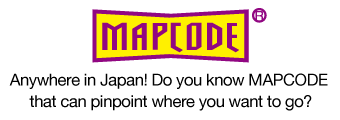###Definition and principle of MAPCODE
• 1) Definition of MAPCODE
MAPCODE divides the whole of Japan into meshes. MAPCODE is defined as uniform expression of each mesh and its relative position within a mesh.
• 2) Code system
MAPCODE uses a standard code system or a high resolution code system in accordance with use purposes of users.
• ■ Standard MAPCODE
Minimum unit of Standard MAPCODE is a rectangle whose length of each side is 1 second in the latitude and longitude, and MAPCODE consists of a maximum of 10 digits. The code is composed of 3 meshes (zone, block and unit).
• ■ MAPCODE HR (high resolution MAPCODE)
Minimum unit of MAPCODE HR is a rectangle whose length of each side is 1/9 seconds. In this case, MAPCODE consists of a maximum of 12 digits with “* (asterisk)”.• ■ Distinguishment of codes
Standard MAPCODE can be distinguished from MAPCODE HR, depending on the presence of “* (asterisk)”. If the MAPCODE has “*”, the MAPCODE is MAPCODE HR.
• 3) Principle of MAPCODEJapan is divided into meshes (zones), whose length of each side is 900 seconds. 1,162 Zones cover the whole of Japan including islands.zone
Define Japan with 1162 zones Use a table that defines the latitude and longitude at the lower left of the zone.block
There are 900 blocks in each zoneunit
There are 900 units in each block.core
There are 9 cores in each unit.S core
There are 9 S cores in each core.• 4) Assignment method of each mesh number
• (1) Zone
Each zone is assigned a number between 0 to 1,162 in accordance with the frequency of use (weighing by population density and road density).
** For more detail on how to set a zone number, please refer to “About the setting method of zone number”
• (2) Block
For 900 blocks in a zone, a number between 0 to 899 is assigned to each block from lower left to upper right, giving priority to the horizontal direction.
• (3) Unit
For 900 units in a block, a number between 0 to 899 is assigned to each unit from lower left to upper right, giving priority to the horizontal direction.
• (4) Core
For 9 cores in a unit, a number between 0 to 8 is assigned to each core as seen in the diagram below. The number 0 is assigned to the core in the center of a unit.
• (5) S core
For 9 S core in a core, a number between 0 to 8 is assigned to each S core as seen in the diagram below. The number 0 is assigned to the S core in the center of a core.
•